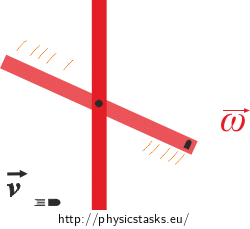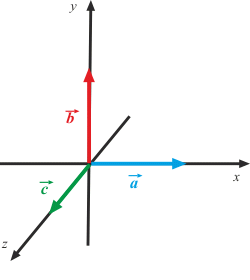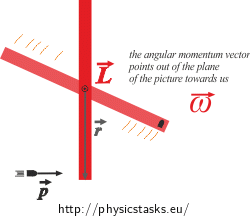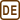## A Projectile Hitting an End of a Rotatable Rod

A wooden rod of length of 40 cm and mass of 1 kg can rotate about the axis perpendicular to the rod and passing through the center of the rod. One end of the rod gets hit by a projectile of mass of 10 g at the speed of 200 m·s-1 in the direction perpendicular to both the axis and the rod. Determine the angular speed of the rod after the projectile gets stuck in it.

Note: We assume that the projectile first very quickly buries into the rod and then the system starts moving with the angular speed we are solving for.• #### Notation

 d = 40 cm length of the rod M = 1 kg mass of the rod m = 10 g mass of the projectile v1 = 200 m·s−1 speed of the projectile ω = ? (s−1) angular speed of the rod
• #### Theory – Cross Product

By the cross product of vectors $$\vec{a}\times \vec{b}$$ we understand an operation that to the two vectors assigns a vector $$\vec{c}$$ which satisfies:

• $$\vec{c} = \vec{n} |\vec{a}||\vec{b}| sin \varphi$$
• Vectors $$\vec{a}\vec{b}\vec{c}$$ form a right-handed basis

Vector $$\vec{n}$$ is a unit vector perpendicular to both the vectors $$\vec{a}$$ and $$\vec{b}$$, φ is the angle formed by the two vectors $$\vec{a}$$ and $$\vec{b}$$.

Whether the vectors $$\vec{a}\vec{b}\vec{c}$$, where $$\vec{c}$$ is perpendicular to the other two, form a right-handed basis can be determined using the right-hand rule. If the fingers of your right hand show the direction of the shortest way the two vectors $$\vec{a}$$ and $$\vec{b}$$ can be overlapped then your raised thumb shows the direction of the vector $$\vec{c}$$. If that is not the case the basis is left-handed.

These are some important equalities of the cross product:

• φ = 0 (the vectors are parallel) → $$\vec{a}\times \vec{b} = 0,$$
• φ = π/2 or 3π/2 (the vectors are perpendicular) → $$\vec{a}\times \vec{b} = \vec{n} |\vec{a}||\vec{b}|,$$$\vec{a}\times \vec{b} = \vec{c},$ $\vec{b}\times \vec{a} = -\vec{c},$ $\vec{c}\times \vec{a} = \vec{b},$ $\vec{c}\times \vec{b} = -\vec{a}.$

And so on.

• #### Hint 1

What applies to the system of the rod and the projectile before and after the projectile hits the rod? Is there a conserved quantity?

• #### Hint 2

What is the definition of angular momentum? Write its definition for a mass point and the formula you can use in the case of a rigid body rotating about a fixed axis.

Also write the respective magnitudes of the angular momentum vectors and think about their direction.

• #### Hint 3

Write down what the angular momentum of the rod-projectile system is equal to before and after the impact. Find its direction and write the respective lengths of the angular momentum vectors for both situations.

• #### Hint 4

Use the law of conservation of angular momentum to solve for the angular velocity.

• #### Total SolutionWhen solving the assignment we will start with the law of conservation of angular momentum. We will look at situation (1) right before the projectile hits the rod – a point at which the projectile starts moving along a circular trajectory – and situation (2) right after the impact when the projectile and the rod start moving together.

From the law of conservation of angular momentum we get:

$\vec{L}_1 = \vec{L}_2\,,$

where

$$\vec{L}_1$$ ... net angular momentum of the rod-projectile system right before the impact,

$$\vec{L}_2$$ ... net angular momentum of the rod-projectile system right after the impact..

Situation (1) – Right before the impact:

In this situation the rod isn’t moving, the total angular momentum is therefore equal to the angular momenum of the projectile.

Angular momentum of the projectile with respect to the axis of rotation:

$\vec{L}_1 = \vec{r} \times \vec{p}\,.$

The vector is perpendicular to the screen (paper) and points towards us:

The magnitude of the vector is:

$L_1 = rp\sin\varphi \,,$

where φ is equal to 90° and r is equal to d/2.

$L_1 = pr = mvr = \frac{1}{2}mdv\tag{1}$

Situation (2) – right after the impact:

The projectile had already burried into the rod and they started rotating. For the angular momentum we can write:

$\vec{L}_2 = I\vec{\omega}\,,$

where I is the moment of inertia of the rod and the projectile with respect to the axis of rotation that passes through the center of the rod and $$\vec{\omega}$$ is the angular velocity of the rotation we are solving for.

For the magnitude of the angular momentum vector it holds:

$L = I\omega\,.$

The direction of the angular momentum vector is given by the direction of the angular velocity vector and like the axis of rotation it is perpendicular to the rod and like the vector $$\vec{L}_1$$ it points outside of the screen (paper).

The moment of inertia of a thin rod rotating about its center and the moment of inertia of a mass point (which we will use as an approximation for the projectile) rotating on a circle with diameter d are:

$I_{rod} = \frac{1}{12}Md^2\,,$ $I_{projectile} = \frac{1}{4}md^2\,.$

From that we can get the magnitude of the angular momentum vector in situation 2:

$L_2 = \omega(I_{rod} + I_{projectile})\,,$ $L_2= \omega d^2(\frac{1}{12}M + \frac{1}{4}m)\,.\tag{2}$

From the law of conservation of angular momentum we get:

$\vec{L}_1 = \vec{L}_2\,.$

The equality also holds for the magnitudes of the angular momentum vectors.

$L_1 = L_2\,.$

We substitute from (1) and (2):

$\frac{1}{2}mdv = \omega d^2(\frac{1}{12}M + \frac{1}{4}m)\,.$

We get:

$\omega = \frac{mv}{d(\frac{1}{6}M+\frac{1}{2}m)}\,,$
$\omega = \frac{0.01 {\cdot} 200}{0.4 \cdot (\frac{1}{6} \cdot 1+\frac{1}{2} \cdot 0.01)} s^{-1} = 29.1 s^{-1}\,.$
$\omega = \frac{mv}{d(\frac{1}{6}M+\frac{1}{2}m)}\,.$